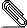[NTG-context] mathring circle above a letter

Hi all,

The \mathring command puts a small circle above a letter, but in some fonts
(namely Lucida OT) that circle is too small. So thanks to a hint by Hans and
Wolfgang some years ago I use the following definition which works fine when
used alone. But in maths, one needs sometimes to use it with the command
\overline (or \overbar) and the result is not nice.

Can someone tell me please whether it is possible to modify the \mathring
command so that th ecircle is a little bit larger, or the one I define below as
\interior in order to have a correct result (more clearly said: I would like
the small circle to be put above the bar which is itself above the A in
$\interior{\overline{A}}$).

Thanks for any help: OK

%%% begin mathring-interior.tex
\setuppapersize[A5][A5]
\definemathstackers[MySymbol]
[voffset=-1.2\mathexheight,
hoffset=\zeropoint,
mathclass=ord,
topoffset=.3\mathemwidth, % poor man's italic correction
middlecommand=\mathematics]
%\define\interior{\mathover[MySymbol]{"2218}{#1}} %2218 U+00B0
\define\interior{\mathover[MySymbol]{176}{#1}} %2218 U+00B0

\starttext
The interior of a set $A$ is denoted by $\interior{A}$.

The interior of a set $A$ is denoted by $\mathring{A}$.

The closure of the interior is $\overline{\interior{A}}$.

Beware $\overline{\interior{A}} \neq \interior{\overline{A}}$. (This is not
nice\dots).

Beware $\overline{\mathring{A}} \neq \mathring{\overline{A}}$
\stoptext

%%% end mathring-interior.texmathring-interior.pdf
Description: Adobe PDF document

___________________________________________________________________________________
If your question is of interest to others as well, please add an entry to the
Wiki!

maillist : ntg-context@ntg.nl / http://www.ntg.nl/mailman/listinfo/ntg-context
webpage  : http://www.pragma-ade.nl / http://context.aanhet.net
archive  : https://bitbucket.org/phg/context-mirror/commits/
wiki     : http://contextgarden.net
___________________________________________________________________________________